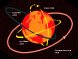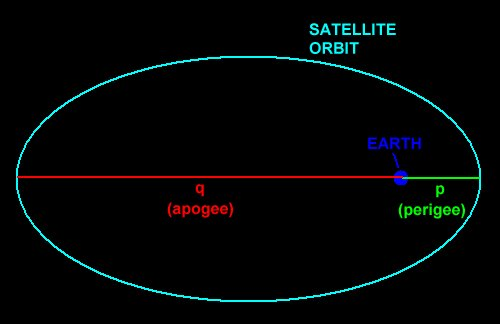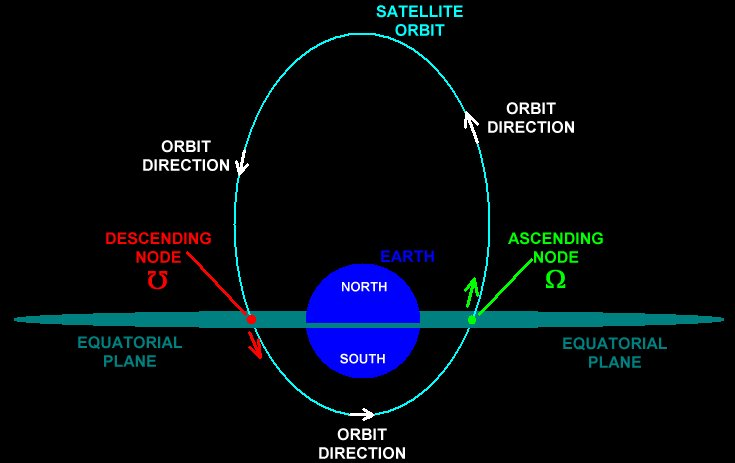Apogee (q) refers to the furthest distance that the satellite can have from the Earth. It can also refer to that point in the satellite's orbit at which the satellites reaches the furthest distance.

Perigee (p) refers to the closest distance that the satellite can have from the Earth. It can also refer to that point in the satellite's orbit at which the satellites reaches the closest distance.Semi-Major Axis (a): The distance from the center of the orbit ellipse to satellite's apogee or perigee point. This is also defined as the average distance of the satellite from the Earth's center.

Semi-Minor Axis (b): The shortest distance from the true center of the orbit ellipse to the orbit path.

Focus Distance (c): The distance from the true center of the orbit ellipse to the center of the Earth.Period (T): The time required for the satellite to orbit the Earth once.

Ascending Node (W): The precise point in a satellite's orbit that intersects the equatorial plane of the Earth as the satellite moves from the southern to the northern hemisphere (ascending).

Descending Node (Ʊ): The precise point in a satellite's orbit that intersects the equatorial plane of the Earth as the satellite moves from the northern to the southern hemisphere (descending).